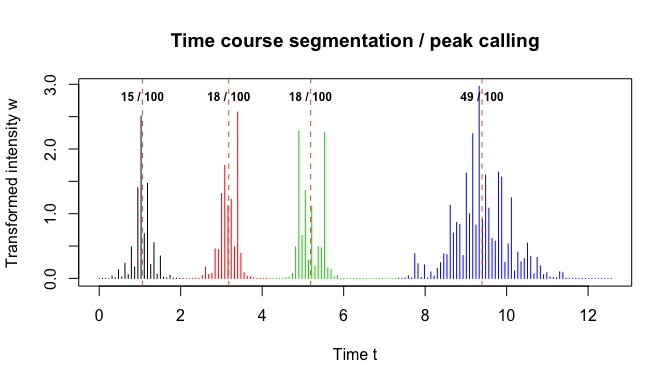# Tutorial: Optimal univariate clustering

#### 2019-09-06

This tutorial illustrates applications of optimal univariate clustering function Ckmeans.1d.dp. It clusters univariate data given the number of clusters $$k$$. It can estimate $$k$$ if not provided. It can also perform optimal weighted clustering when a weight vector is provided with the input univariate data. Weighted clustering can be used to analyze 1-D signals such as time series data. The corresponding clusters obtained from weighted clustering can be the basis for optimal time course segmentation or optimal peak calling.

## 1. Cluster univariate data given the number of clusters $$k$$

### Example 1.

Cluster data generated from a Gaussian mixture model of three components.

The number of clusters is provided.

require(Ckmeans.1d.dp)
## Loading required package: Ckmeans.1d.dp
x <- c(rnorm(50, sd=0.3), rnorm(50, mean=1, sd=0.3), rnorm(50, mean=2, sd=0.3))
# Divide x into 3 clusters
k <- 3
result <- Ckmeans.1d.dp(x, k)
plot(result)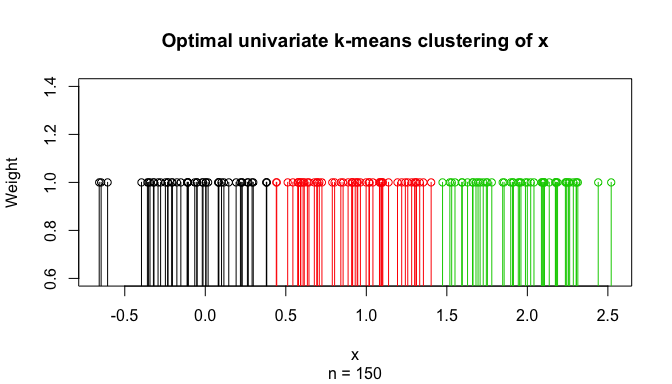plot(x, col=result$cluster, pch=result$cluster, cex=1.5,
main="Optimal univariate clustering given k",
sub=paste("Number of clusters given:", k))
abline(h=result$centers, col=1:k, lty="dashed", lwd=2) legend("bottomright", paste("Cluster", 1:k), col=1:k, pch=1:k, cex=1.5, bty="n")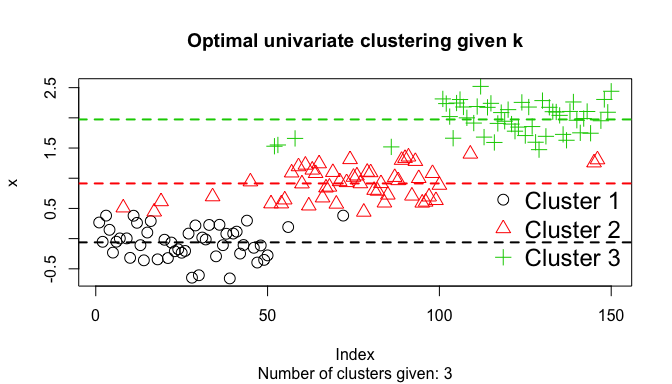## 2. Cluster univariate data with an estimated number of clusters $$k$$ ### Example 2. Cluster data generated from a Gaussian mixture model of three components. The number of clusters is determined by Bayesian information criterion: require(Ckmeans.1d.dp) x <- c(rnorm(50, mean=-1, sd=0.3), rnorm(50, mean=1, sd=1), rnorm(50, mean=2, sd=0.4)) # Divide x into k clusters, k automatically selected (default: 1~9) result <- Ckmeans.1d.dp(x) plot(result)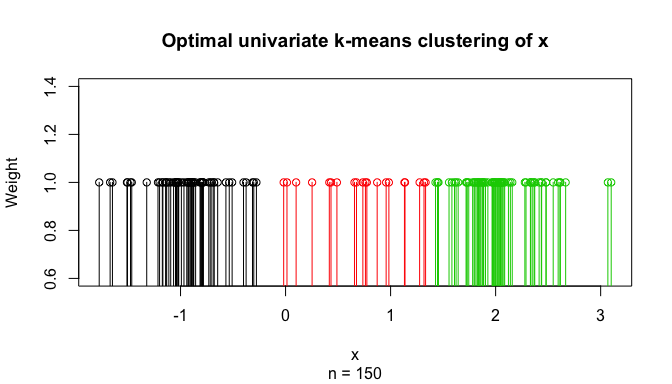k <- max(result$cluster)
plot(x, col=result$cluster, pch=result$cluster, cex=1.5,
main="Optimal univariate clustering with k estimated",
sub=paste("Number of clusters is estimated to be", k))
abline(h=result$centers, col=1:k, lty="dashed", lwd=2) legend("topleft", paste("Cluster", 1:k), col=1:k, pch=1:k, cex=1.5, bty="n")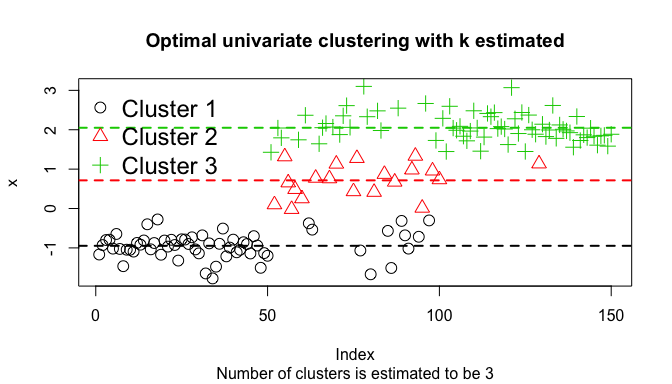## 3. Optimal weighted univariate clustering for time series analysis ### Example 3. We segment a time course to identify peaks using weighted clustering. The input data is the time stamp of obtaining each intensity measurement; the weight is the signal intensity. require(Ckmeans.1d.dp) n <- 160 t <- seq(0, 2*pi*2, length=n) n1 <- 1:(n/2) n2 <- (max(n1)+1):n y1 <- abs(sin(1.5*t[n1]) + 0.1*rnorm(length(n1))) y2 <- abs(sin(0.5*t[n2]) + 0.1*rnorm(length(n2))) y <- c(y1, y2) w <- y^8 # stress the peaks res <- Ckmeans.1d.dp(t, k=c(1:10), w) plot(res)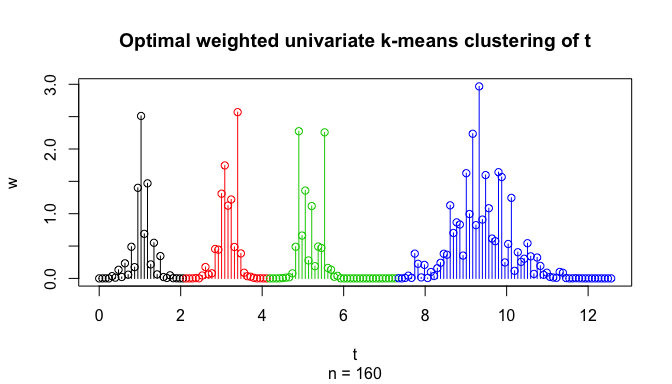plot(t, w, main = "Time course segmentation / peak calling", col=res$cluster, pch=res$cluster, type="h", xlab="Time t", ylab="Transformed intensity w") abline(v=res$centers, col="chocolate", lty="dashed")
text(res$centers, max(w) * .95, cex=0.75, font=2, paste(round(res$size / sum(res\$size) * 100), "/ 100"))